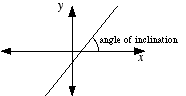index: click on a letter A B C D E F G H I J K L M N O P Q R S T U V W X Y Z A to Z index index: subject areas numbers & symbols sets, logic, proofs geometry algebra trigonometry advanced algebra & pre-calculus calculus advanced topics probability & statistics real world applications multimedia entrieswww.mathwords.com about mathwords website feedback

 Angle of Inclination of a Line The angle between a line and the x-axis. This angle is always between 0° and 180°, and is measured counterclockwise from the part of the x-axis to the right of the line. Note: All horizontal lines have angle of inclination 0°. All vertical lines have angle of inclination 90°. Also, the slope of a line is given by the tangent of the angle of inclination.